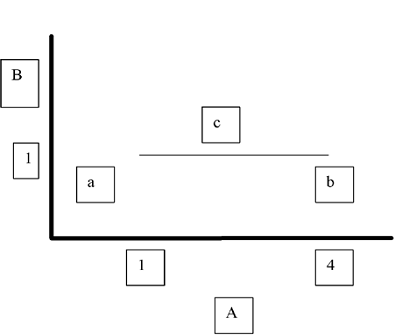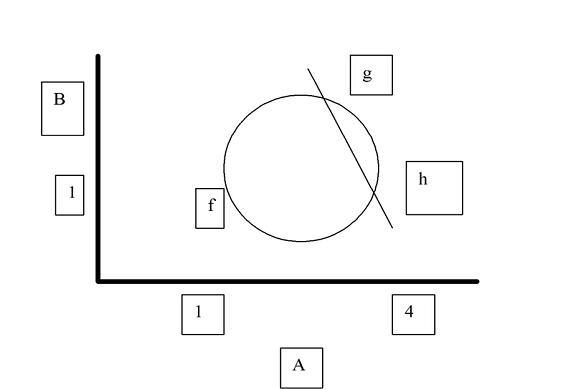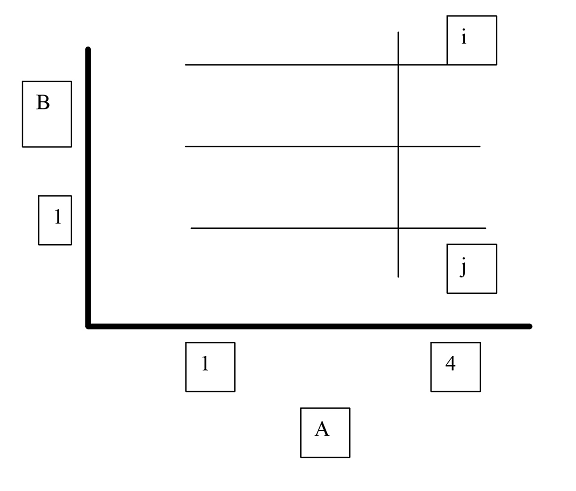Limits, off topic:
Many years ago, as a freshman, I took a college calculus course.  It was an accelerated course.  I made a B, but I was totally at sea throughout.  As the professor developed the technique he used English letters for variables or whatever until they were exhausted, then went to Greek letters and when they were finished started in on Hebrew letters.  One thing that troubled me was the definition of a limit.  Consider a line segment ab in a plane with axes A on the abscissa and B on the ordinate.The value of point a is 1,1, meaning that the value on the abscissa is 1 and the value on the ordinate is 1. The value of b is 4,1.  Well and good; that should be simple enough.

Now take a point c that moves along line segment ab.  The value is a, whatever.  As c gets closer to a, c equals 1.  Now let us say that we define d as any number, however small. If for every d we can find a number e, such that if the number c is less than e then the difference between a and 1 will be less than or equal to d, then we say that 1 is the limit of c at a on the abscissa.

Of course that’s trivial.  But look how it works with something a bit more complex.  Take the same plane AB and insert a circle f and a line that cuts across the circle at g and h.The slope of gh changes as h moves.  The slope, of course, is the amount the line goes up or down on the B axis for any movement on the A axis.  If you know the value of g on the A axis along with the center and the radius of the circle it’s pretty straightforward to calculate the value on the B axis, same with h.  So given the A values of g and h you can get the slope.  Now let the A value of h approach the A value of g.  The slope changes.  It gets closer and closer to the slope of a tangent to the circle at g.  But you can’t let it get quite there.  If h equals g, then you have one point, which does not define a line.  But you can get as close as you like.  So the slope of the tangent at g is the limit as h approaches g.  All you have to do is to prove that for any difference between the slope of gh and that limit you can find a value for h that makes the difference between gh and that limiting slope less than the distance you specified.

And that can be done.  Presto, you have the slope of the tangent.

But now consider this: (first of all, you know that some numbers are “rational” in that they can be expressed as a ratio of two integers such as 2 to 3, and some are not, like the square root of 2, for which there are no integers that will give the number.  If you doubt that, drop me a line and I’ll explain in a different post) consider three sets of points on plane ABNow let all the values of one set be 1 if the A value is not rational.  Let all the values of another set be 2 if the number comes out even in decimal.  Let the third set be everything else on the A axis all equal to three.

Consider a vertical line ij through the line sets.  Now as the A value of the intersection of line ij approaches 1, what is the limit?  Well, it could be 1, 2 or 3, couldn’t it?  I mean for an number however small you can find a value for the line on the A axis such that the difference between the B value of the intersection with the lowest set and 1 is less than your test number.  The same goes for 2 and 3.  So there are three values, or as many as you care to set up, for the limit of the B value at 1, or anywhere else, for that matter.

I probably messed that up.  But if you were going to muddle through it all, you probably see what I am saying.  It has troubled me for decades.  But I was reading about Newton for a project I hope to unveil for you some day and it finally struck me.

There have been 86 visitors over the past month.